###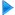HF Antennas

Horizontal dipole

Invelco AT-110 folded dipole

Marconi

Monopoles - Ground planes

Multiband multi-dipole

Rhombic antennas

Sloping Vee 470

Windom

###Other resources

HF Antennas

HF Central

Technical Articles

VHF-UHF Central

EA4FSI-28T1 Home# Rhombic AntennasEste artículo también está disponible en Español (this article is also available in Spanish).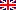Abstract:

Description and simulation of two types of rhombic antennas, using the software 4Nec2: the simple bi-directional and the terminated directional rhombic antenna. This kind of antennas is well known thanks to their relative high gain associated to a simple design, being the main drawback the formation of important secondary lobes in the radiation pattern. The analysis takes into account the variations of several parameters, such as the size of the antenna, the acute angle of the rhombus, the value of the resistive load (in the terminated case) and the height of installation.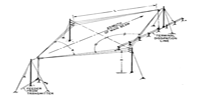Type: Progressive wave Design: Impedance: 300 ohms Simulation: 4Nec2 Band: Broadband Remarks: Broadband antenna from the SWR point of view. Design for the 20 m band.

# 1. Rhombic antenna.

In this section we will consider the design and simulation of a rhombic antenna terminated in open circuit, without loads of any kind. As we will see, the result will be a bi-directional antenna.

## 1.1. Design of the antenna.

The rhombic antennas are made of two acute-angle V-beams placed end-to-end, terminated in an open circuit. Each side of the antenna is made of two legs of length "L" and as a whole the antenna has the shape of a rhombus, that is, the opposite angles are of the same value (fig.1).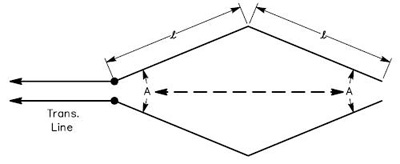Fig.1. Rhombic antenna. The ARRL Antenna Book, Chapter13, 20th Ed.

As we will see later, the value of "L" must be an integral number of half wavelengths at the frequency of design. The antenna is feeded at the opposite side of the open circuit.

## 1.2. Antenna modeling using 4Nec2.

In order to simulate the antenna in 4Nec2, we will use the draw shown in the fig.2. The feeding point and the opposite open circuit will be placed in the Y-axis, whereas the other two angles of the antenna will be placed in the X-axis.

We will use the following variables:

• L = leg length (meters).

• A = acute angle of rhombus (degrees).

• H = height of installation (meters).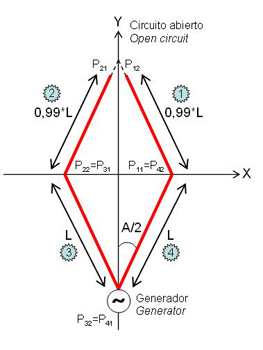Fig.2. Model of the rhombic antenna.

We will define four legs as shown in the figure, whose bounds are the following points (P) with their corresponding coordinates:

• Leg #1: P11 (x11, y11) - P12 (x12, y12).

• Leg #2: P21 (x21, y21) - P22 (x22, y22).

• Leg #3: P31 (x31, y31) - P32 (x32, y32).

• Leg #4: P41 (x41, y41) - P42 (x42, y42).

The legs #1 and #2 are reduced 1% in order for this side to become an open circuit.

Using trigonometry, the coordinates of the points delimitating the leg #1 will be: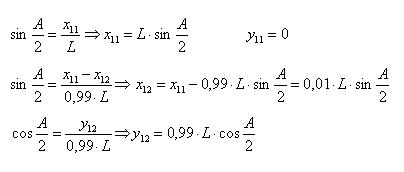The coordinates for the leg #2 are::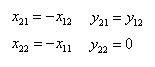Using trigonometry again, the coordinates for the leg #3 will be: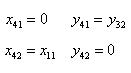And finally, the coordinates for the leg #4 are::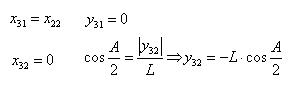We will suppose a frequency of design of 14 MHz (21.42 m wavelength). Lets recall that "L" must be an integral number of half wavelengths at the frequency of design. We will choose then, i.e. L=21.42 m and A=62º. With those parameters and a scenario of free space, the antenna model in 4Nec2 is shown in the fig.3.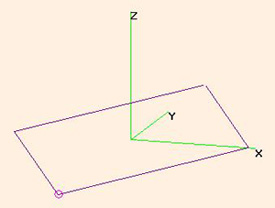Fig.3. Rhombic antenna model in 4Nec2.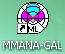Antena_Rombica.nec

## 1.3. Standing wave ratios.

Having the variables initialized according to the values shown in the previous section, at a frequency of 14 MHz the antenna has a SWR=13.2 for a characteristic impedance of 300 ohms.Fig.4. SWR @ 300 ohms for a rhombic antenna in free space, L=21,42 m and A=62º.

The fig.4 shows the simulation results for all the HF band (3-30 MHz), using the same parameters. We can see that, in most of the HF band, the antenna could be easily tuned. The SWR is lower at the highest frequencies.

The fig.5 shows the radiation pattern of the same antenna in free space, at a frequency of 14 MHz.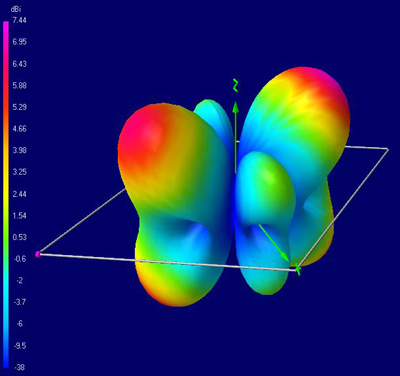Fig.5. Rhombic antenna in free space, F=14 MHz, L=21,42 m and A=62º. 3D radiation pattern.

The antenna is bi-directional, having two main lobes along the Y-axis and two secondary lobes along the X-axis. The maximum gain is 7.44 dBi. One peculiarity of the antenna is that its main lobes are quite elevated, 45º in this case.# 2. Terminated rhombic antenna.

In order to achieve a directive antenna, we will replace the open circuit with a resistive load. In this section we will analyse a terminated rhombic antenna designed for the 14 MHz band.

## 2.1. Design of the antenna.

The fig.6 shows the classical design of an horizontal terminated rhombic antenna with three wires, used to achieve a wider working band. The resistive load has usually a value between 600 and 800 ohms.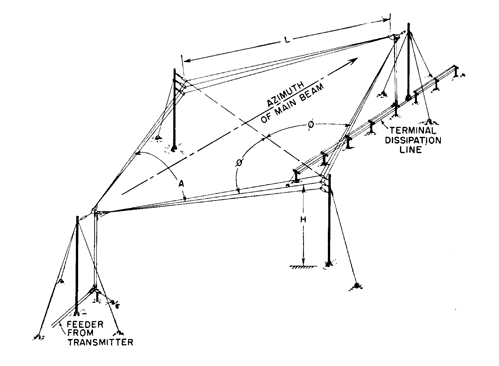Fig.6. 3-wire horizontal terminated rhombic antenna. Radio Antenna Engineering, p.316 (Laport, 1952).

The fig.7 shows a graph to calculate the optimum parameters for this antenna, taking as a goal the maximum gain and the minimum amplitude of the secondary lobes. As we can see, the leg length, the acute angle of the rhombus, the height of installation, the elevation of the main beam and the beamwidth are closely related.Fig.7. Optimum parameters. Radio Antenna Engineering, p.321 (Laport, 1952).

Remark: the height of the rombus is shown in feet.

In our example, however, we will consider the simplest case, with each side of the antenna made with only one wire.

## 2.2. Antenna modeling using 4Nec2.

In order to design the terminated rhombic antenna in 4Nec2, we will use the same model shown in the section 1.2 (fig.2), adding one more leg to close the open circuit between legs #1 and #2. The resistive load will be placed in this new leg.

We will use the following variables:

• R = load resistance (ohms).

• L = leg length (meters).

• A = acute angle of rhombus (degrees).

• H = height of installation (meters).

The 4Nec2 antenna model is shown in the fig.8.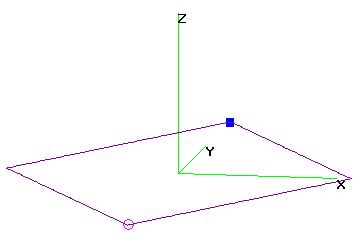Fig.8. Terminated rhombic antenna model in 4Nec2.Antena_Rombica_Carga.nec

## 2.3. Standing wave ratios.

Having the variables initialized according to the values shown in the previous sections and using a load R=600 ohms, at a frequency of 14 MHz the antenna has a SWR=2.4 for a characteristic impedance of 300 ohms.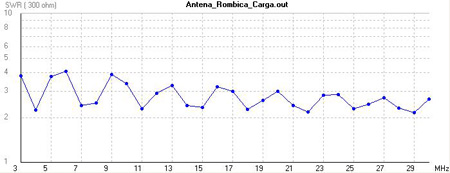Fig.9. SWR @ 300 ohms for a terminated rhombic antenna in free space, L=21,42 m and A=62º.

The fig.9 shows the simulation results for all the HF band (3-30 MHz), using the same parameters. We can see that the SWR is markedly lower than in the previous case without the resistive load (fig.4). This improvement is more remarkable at the lowest frequencies.

The fig.10 shows the radiation pattern of the same antenna in free space, at a frequency of 14 MHz.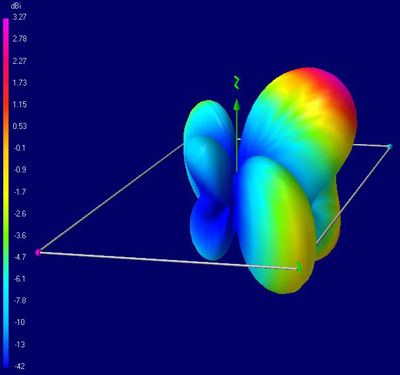Fig.10. Terminated rhombic antenna, free space, F=14 MHz, L=21,42 m, A=62º. 3D radiation pattern.

The main effect of terminating the antenna with a resistive load is that the radiation pattern is no more bi-directional, being now directional with a lower maximum gain (3.27 dBi vs 7.44 dBi of the antenna without load). This is due to the load disipating around half of the power of the transmitter. The main lobe is placed in the positive Y-axis, with an elevation of 40º.

Although we have achieved a directional pattern, due to the size and construction of the antenna it should be almost impossible to rotate, so its use is limited to fixed radio links or SIGINT stations, as was the case of the RAF "Y Stations" during the Second World War.

The fig.11 shows the radiation patterns of the same antenna in free space, at the following frequencies: 3.8 MHz, 7 MHz, 18 MHz and 21 MHz.Fig.11. Radiation patterns at several bands of interest.

At the 3.8 MHz band, the radiation pattern is completely vertical, making the antenna suitable for NVIS. However, the antenna has -6.2 dBi losses at a takeoff angle of 90º.

At the 7 MHz band, the main lobe has an elevation of about 70º. The antenna still has losses, -3 dBi in this case.

At 18 MHz, above the frequency of design (14 MHz), the antenna has no more losses, presenting a gain of 5.6 dBi and a main lobe with a takeoff angle of 35º.

At 21 MHz, the maximum gain is 6.7 dBi and the main lobe has an elevation of 30º.

We can conclude that, although the impedance of the antenna varies slightly all along the HF band, the radiation patterns are heavily frequency dependant. In practice, the design for an optimal main lobe and reduced secondary lobes can be achieved for a relatively narrow badwidth around the frequency of design.

## 2.5. Effects of the variation of the design parameters.

In this section we will analyze the possible effects of varying the parameters of design of the antenna: load resistance (R), length (L), acute angle of rhombus (A) and height of installation (H).

We will analyze the changes using several different resistive loads:

• F = 14 MHz.

• R: 300, 600 and 900 ohms.

• L = 21.42 m.

• A = 62º.

• H: does not have influence (free space).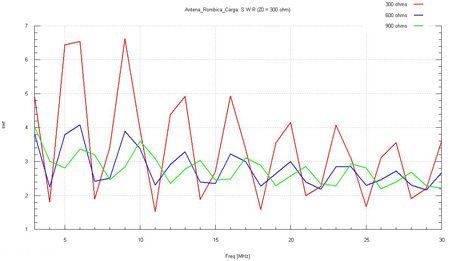Fig.12. SWR comparison @ 300 ohms in all the HF band for loads of 300, 600 and 900 ohms.

The fig.12 shows the SWR calculated in all the HF band, using loads of 300, 600 and 900 ohms, for a characteristic impedance of 300 ohms. Click in the image to see a larger version. We can see the following:

• 300 ohms load: the SWR (1.88) is the best at the frequency of design (14 MHz), but it is quite high in several bands, especially in the lower ones, reaching values up to 6.61.

• 600 ohms load: the SWR gets worse at the frequency of design (2.40), but is remarkably lower in all the bands, dropping as the frequency increases.

• 900 ohms load: at the frequency of design, the SWR is slightly worse than in the previous case (3.03), but if we consider all the HF band, it is undoubtedly the better case, being the highest SWR equal to 4.02.

The fig.13 shows a comparison of the antenna gain in all the HF band, using loads of 300, 600 and 900 ohms. The values are measured in the direction of maximum radiation, which in all the cases corresponds to the positive Y-axis and a takeoff angle of 45 degress. Click on the image to see a larger version.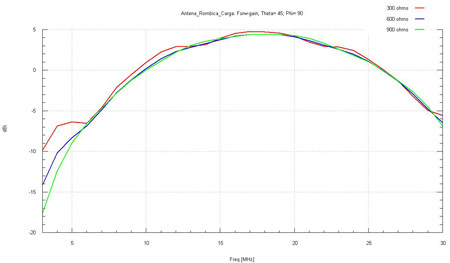Fig.13. Comparison of the antenna gain in the HF band, using loads of 300, 600 and 900 ohms.

We can see that the value of the load resistance does not have impact in the antenna gain, which on the other hand presents three different zones:

• Between 3 MHz and around 9 MHz, the antena has losses.

• Between around 9 MHz and 26 MHz, the antenna hasn't losses, being the maximum gain of 4.75 dBi at 17 MHz.

• Between 26 MHz and 30 MHz, the antenna has losses again.

### 2.5.2. Leg length.

We will analyze the changes using several different leg lengths "L", that is, we will check how the size of the antenna has an influence on the SWR and the radiation pattern for a specific frequency of design:

• F = 14 MHz.

• R = 600 ohms.

• L: sweep between half wavelength (10.71 m) and three wavelengths (64.28 m).

• A = 62º.

• H: does not have influence (free space).Fig.14. SWR comparison @ 300 ohms at 14 MHz for different values of L.

The fig.4 shows the SWR calculations for a characteristic impedance of 300 ohms, at a frequency of 14 MHz and for different lengths L between half wavelength and three wavelengths. See how the minimum SWR values are achieved when L is an integral number of half wavelengths at the frequency of design.

The table 1 shows the maximum gain values for each L value equal to an integral number of half wavelengths (lambda) at the frequency of design. The takeoff angle of the main lobe of the radiation pattern is also shown.

L (0.5 x lambda) L (m) Gain (dBi) Takeoff angle (º)
x 1 10.71 - 3 70
x 2 21.42 3.27 40
x 3 32.14 6.54 30
x 4 42.85 8.74 20
x 5 53.57 10.42 10
x 6 64.28 11.65 0

Table 1. Antenna gain and takeoff angle for different values of L.

We can conclude that the antenna must be designed with a length "L" equal to an integral number of half wavelengths at the frequency of design, in order to achieve the best SWR. Within those possible values, the higher ones will provide higher antenna gains and lower takeoff angles.

### 2.5.3. Acute angle of rhombus.

In this section we will study the changes resulting of variations of the acute angle of the rhombus, "A", keeping constant the size of the antenna:

• F = 14 MHz.

• R = 600 ohms.

• L = 21.42 m.

• A: sweep between 30º and 90º.

• H: does not have influence (free space).

The video 1 shows the results of the simulated 3D patterns. We can see that the main effect of the variations of the angle "A" is the creation of secondary lobes in the radiation pattern, shifted 90º from the direction of maximum radiation. The forward gain also changes. In a directional antenna, those secondary lobes may cause a worse signal to noise ratio in the radio communications.

Video 1. Variations of the forward gain and secondary lobes as a result of
changing the acute angle of rhombus

En la fig.15 se muestran la ganancia delantera de la antena (verde) y la relación entre la ganancia del lóbulo principal y la ganancia de los lóbulos secundarios que aparecen a 90 grados (rojo). Ambos parámetros están medidos a una elevación de 45 grados, haciendo variar el valor del ángulo "A" de la antena entre 30 grados y 60 grados. Pulse en la imagen para verla a tamaño completo.

The fig.15 shows the plot of the forward gain (green) and the ratio between the gain of the main lobe and the gain of the secondary lobes at 90º (red). Both parameters are measured at a takeoff angle of 45º. The angle "A" varies between 30º and 90ºFig.15. Antenna gain and comparison between lobes for different values of "A".

For small values of the angle "A", the secondary lobes are quite small, a fact quite interesting for a directional antenna. With A=30º, the ratio between the main lobe and the secondary lobes is 17 dB. However, the antenna has losses of 2 dBi in the forward direction.

On the other hand, if we select A=90º (square antenna), the forward gain will be the best (around 4.5 dBi), but the ratio between lobes is the worst (4.5 dB).

We can see that, for values around A=60º, the antenna has a forward gain of 3 dBi and the ratio between lobes is 9 dB, so we can consider this case as optimal.

In regards of the SWR, the simulation shows that this parameter is scarcely related with the variations of "A".

### 2.5.4. Height of installation.

In this section we will analyze the variations as a result of changing the height of installation "H" of the antenna. To achieve this, we will place the antenna parallel to a perfect ground plane.

• F = 14 MHz.

• R = 600 ohms.

• L = 21.42 m.

• A = 62º..

• H: sweep between 2.5 m and 53.5 m.

The video 2 shows that the main effect of this change is a variation of the elevation of the main lobe in the radiation pattern. Significant secondary lobes are created.

Vídeo 2. Variations in the vertical radiation pattern as a result of changing the height of installation

En la tabla 2 se muestran, para valores "H" de interés, las amplitudes relativas de los lóbulos principal y secundarios del diagrama de radiación vertical, así como su elevación.

The table 2 shows, for some selected values of "H", the relative amplitudes of the main and secondary lobes, as well as their takeoff angle.

Height H (m) Main lobe Secondary lobes
Gain (dBi) Takeoff angle (º) Gain (dBi) Takeoff angle (º)
2.5 3.83 50 N/A N/A
5.5 8.27 45 N/A N/A
8.6 8.98 40 N/A N/A
10.7 (*) 8.63 35 N/A N/A
11.2 8.48 35 N/A N/A
11.7 8.26 32 -19.00 75
14.2 7.57 25 -0.14 65
17.3 6.73 20 6.47 55
21.4 (*) 8.87 45 5.85 15
24.4 9.27 40 5.55 15
27.5 8.98 35 5.11 / 2.61 10 / 60
30.6 8.15 30 6.69 / 5.19 55 / 10
32.1 (*) 8.37 30 7.79 / 5.16 55 / 10
33.6 8.43 50 8.17 / 5.08 30 / 10
36.7 9.03 45 7.52 / 4.81 25 / 10
39.2 8.93 45 7.55 / 4.39 / 2.60 25 / 10 / 65
42.8 (*) 9.07 40 6.29 / 5.99 / 3.66 60 / 25 / 5
45.9 8.89 35 7.92 / 6.71 / 3.98 55 / 20 / 5
48.4 8.80 50 8.61 / 6.69 / 4.21 35 / 20 / 5
51.5 8.82 45 7.99 / 5.86 / 4.42 30 / 20 / 5
53.5 (*) 9.22 45 8.39 / 5.01 / 4.74 30 / 60 / 20

Table 2. Gain and takeoff angle of the radiation lobes for selected values of H.

The marked values (*) are multiples of half wavelength at the frequency of design.

We can see that the general trend is: for higher heights H, higher values of the antenna gain, although the takeoff angle of the main radiation lobe will vary.

Up to a height of 11.2 m, there aren't any secondary lobes. From this height on, a secondary lobe is created, which at 14.2 m is 7 dB below the main lobe and at 24.4 m is only 3.7 dB below. From 27.5 m on, a new significant secondary lobe is created. From 33.6 m on, this secondary lobe becomes the main lobe. Finally, from 39.2 m on, there are three significant secondary lobes.

Checking all the table, if the takeoff angle of the radiation pattern is a requirement of design, taking into account all the possible lobes we can cover the whole range between 5º and 60º.# 3. Conclusions.

The rhombic antenna is a wideband progressive wave antenna, made of two acute-angle V-beams placed end-to-end and terminated in an open circuit or in a resistive load. Each side of the antenna is made of two legs of length "L" and as a whole the antenna has the shape of a rhombus, that is, the opposite angles are of the same value.

The non-terminated rhombic antenna is bi-directional and presents an acceptable SWR in all the HF band, whereas the terminated rhombic antenna is directional and improves the SWR, but has a worse gain than the previous one. In both cases, the antenna impedance is close to 300 ohms.

Although the antenna has the important advantage of presenting an impedance with slight variations in all the HF band, the radiation patterns are highly frequency dependant, showing changes in the gain, the takeoff angle of the main lobe and the number of secondary lobes, which in some cases may present significant amplitudes.

Several simulations have been performed, taking into account the following parameters of design:

• F: frequency of design (MHz).

• L: leg length(m).

• A: acute angle of rhombus (degrees).

• H: height of installation (m).

The table 3 shows the effects of all those parameters in the design of the antenna.

SWR Gain Takeoff angle Secondary lobes
R Yes No No Rear lobes only
L Yes Yes Yes Yes
A No Yes Yes Yes
H Negligible Yes Yes Yes

Table 3. Effects of the parameters of design for rhombic antennas.

The rhombic antennas can be considered as wideband antennas from the impedance point of view, but the radiation patterns are sharply frequency dependant. Due to those facts, this kind of antennas must be optimized to be used at particular frequencies.

 Ismael Pellejero - EA4FSI Contact form Tweet